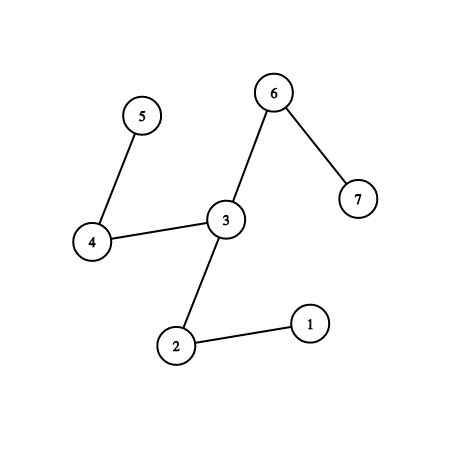시간 제한메모리 제한제출정답맞힌 사람정답 비율
2 초 512 MB279934.615%

## 문제

This is an interactive problem.

Endagorion has a tree on $n$ vertices, and he also showed it to you. He chooses one vertex $u$ as a special vertex, but now he won't tell you anything about it!

Instead, you can ask him questions. For each question, you should choose a vertex $x$, an integer $k$, and $k$ vertices $v_1, v_2, \ldots, v_k$, and he will tell you whether it is true that $\min{(\mathrm{dist}(u, v_i))} \geq \mathrm{dist}(u, x)$. Here, $\mathrm{dist}(p, q)$ is the number of edges in the simple path between vertices $p$ and $q$ in the tree.

You should guess the special vertex using at most $4{\lceil}\log_2{n}{\rceil}$ queries.

Endagorion is very honest, so he wouldn't change the vertex between your queries (in other words, the interactor is not adaptive).

As the constraints are large, and flush is an expensive operation, make sure that you are not flushing too often. You may do it only once after each query.

## 프로토콜

The interaction starts with a line containing one integer $n$: the number of vertices in Endagorion's tree ($2 \le n \le 200\,000$).

Each of the next $n-1$ lines contains two integers $u$ and $v$ ($1 \le u, v \le n$), denoting an edge between $u$ and $v$. It is guaranteed that the given edges form a tree.

After that, you can make queries.

To make a query, print one line containing "? k" ($1 \le k \le n$), an integer $x$ ($1 \leq x \leq n$) and then $k$ distinct integers $v_1, v_2 \ldots, v_k$ ($1 \le v_i \le n$). Separate consecutive integers by single spaces. Then flush the output.

After each query, read one line with a single integer $\mathit{ans} \in \{0,1\}$. If  $\min{(\mathrm{dist}(u, v_i))} \geq \mathrm{dist}(u, x)$, then $\mathit{ans}$ will be equal to $1$. Otherwise, $\mathit{ans}$ will be equal to $0$.

When you find the special vertex $u$ ($1 \leq u \leq n$), print one line containing "! u", and then flush the output and terminate.

Your solution will get Wrong Answer or Time Limit Exceeded if you make more than $4{\lceil}\log_2{n}{\rceil}$ queries.

To flush, you need to do the following right after printing a query and a line break:

• fflush(stdout) or cout.flush() in C++;
• System.out.flush() in Java;
• flush(output) in Pascal;
• stdout.flush() in Python;
• see documentation for other languages.

## 예제 입력 1

5
1 2
1 3
1 4
1 5
1


## 예제 출력 1

? 4 1 2 3 4 5
! 1


## 예제 입력 2

5
1 2
1 3
1 4
1 5
0
0
0
0


## 예제 출력 2

? 4 1 2 3 4 5
? 3 1 2 3 4
? 2 1 2 3
? 1 1 2
! 2


## 예제 입력 3

7
1 2
2 3
3 4
4 5
3 6
6 7
1


## 예제 출력 3

? 3 3 5 7 1
! 3


## 노트## 출처

• 문제를 만든 사람: 300iq

## 채점 및 기타 정보

• 예제는 채점하지 않는다.